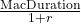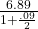IFT Notes for Level I CFA® Program
IFT Notes for Level I CFA® Program

# Part 6

## 5.  Credit and Liquidity Risk

So far in this reading, we focused on how to use duration and convexity to measure the price change given a change in YTM. Now, we will look at what causes the YTM to change.

• The YTM on a corporate bond consists of two parts: government benchmark yield and a spread over the benchmark. Change in YTM can be due to either of these or both.
• The change in the spread can result from a change in credit risk or liquidity risk.
• Credit risk involves the probability of default and degree of recovery if default occurs.
• Liquidity risk refers to the transaction costs associated with selling a bond.
• For a traditional (option-free) fixed rate bond, the same duration and convexity statistics apply if a change occurs in the benchmark yield or a change occurs in the spread.
• A change in benchmark yield could be because of a change in the expected rate of inflation or expected real rate of interest.
• In practice, there often is the interaction between changes in benchmark yields and in the spread over the benchmark, and between credit and liquidity risk. It is rare for any individual component of the YTM to change.

Example 19: Calculating modified duration

Consider a 4-year, 9% coupon paying semi-annual bond with a YTM of 9%. The duration of the bond is 6.89 periods. Calculate the modified duration.

Solution:

Modified duration === 6.593 periods or 3.297 years.

For a one percent change in the annual YTM, the percentage change in the bond price is 3.297%.

Example 20: Calculating the change in the credit spread on a corporate bond

The (flat) price on a fixed-rate corporate bond falls one day from 96.55 to 95.40 per 100 of par value because of poor earnings and an unexpected ratings downgrade of the issuer. The (annual) modified duration for the bond is 5.32. What is the estimated change in the credit spread on the corporate bond, assuming benchmark yields are unchanged?

Solution:

Given that the price falls from 96.55 to 95.40, the percentage price decrease is 1.191%.

-1.191% ≈ -5.32 × ∆Yield,

∆Yield=0.2239%

Given an annual modified duration of 5.32, the change in the yield-to-maturity is 22.39 bps.